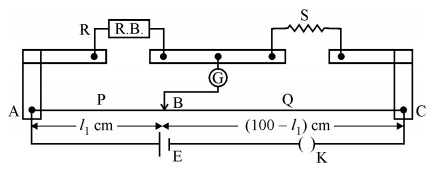# What is end error in a meter bridge? How is it overcome? The resistances in the two arms of the meter bridge areandrespectively. when the resistanceis hunted with an equal resistance the new balance length found to be, whereis the initial balancing length. Calculate the value ofIn the meter bridge, the zero of the ruler doesn't align with the starting end of the wire. This causes a type of zero error known as end error.By using known values ofand, we can determine the end error.

Theoretically, we can calculate the distance fromat which the free end of the galvanometer be connected to get zero deflection.

Experimentally, we can also read the distance on the ruler.

On Substarcting the theoretical value from the reading will give us the zero error.

For meter bridge, applying whetstones bridge principleWe know that,_____(1)

After putting an equal resistance in parallel with S, we have,________(2)

On dividing equation (1) and (2)

We getImpliesOn substituting the value ofin equation (1)

We get## Related Chapters

### Preparation Products

##### Knockout NEET May 2021 (One Month)

An exhaustive E-learning program for the complete preparation of NEET..

₹ 14000/- ₹ 6999/-
##### Foundation 2021 Class 10th Maths

Master Maths with "Foundation course for class 10th" -AI Enabled Personalized Coaching -200+ Video lectures -Chapter-wise tests.

₹ 350/- ₹ 112/-
##### Foundation 2021 Class 9th Maths

Master Maths with "Foundation course for class 9th" -AI Enabled Personalized Coaching -200+ Video lectures -Chapter-wise tests.

₹ 350/- ₹ 112/-
##### Knockout JEE Main April 2021 (One Month)

An exhaustive E-learning program for the complete preparation of JEE Main..

₹ 14000/- ₹ 6999/-